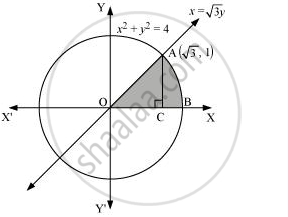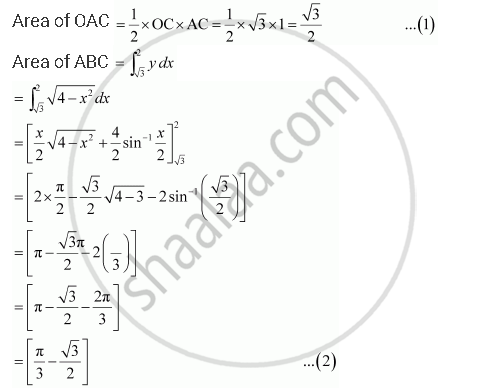Share

Books Shortlist

# Find the Area of the Region in the First Quadrant Enclosed by X-axis, Line X = Sqrt3 Y and the Circle X2 + Y2 = 4. - CBSE (Science) Class 12 - Mathematics

#### Question

Find the area of the region in the first quadrant enclosed by x-axis, line x = sqrt3 y and the circle x2 + y2 = 4.

#### Solution

The area of the region bounded by the circle, x^2 + y^2 = 4, x = sqrt3 and the x-axis is the area OAB.The point of intersection of the line and the circle in the first quadrant is .(sqrt3,1)

Area OAB = Area ΔOCA + Area ACBTherefore, required area enclosed = 32 + π3 - 32 = π3 square units

Is there an error in this question or solution?

#### APPEARS IN

NCERT Solution for Mathematics Textbook for Class 12 (2018 to Current)
Chapter 8: Application of Integrals
Q: 6 | Page no. 366
Solution Find the Area of the Region in the First Quadrant Enclosed by X-axis, Line X = Sqrt3 Y and the Circle X2 + Y2 = 4. Concept: Area Under Simple Curves.
S